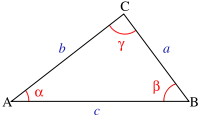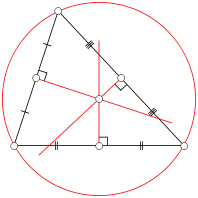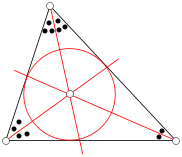Choose language

PL, EN, ES, DE, FR, RU

# Triangle calculator -height, area, perimeter, sides

Triangle calculator will help you calculate the area, perimeter, sides, height, angles of any triangle, right triangle and equilateral.Okrąg opisany Okrąg wpisany

a, b, c - side lengths;
ha, hb, hc - heights from sides a, b, c;
α, β, γ - angles opposite the sides a, b, c;
S - area;
R - radius of the circumscribed circle;
r - radius of the inscribed circle;
p - half the perimeter of the triangle;

## Triangle - Area from sides and height

$$\Large{S=\frac{ah_a}{2}= \frac{bh_b}{2}=\frac{ch_c}{2} }$$
Side length a, b or c

Height to the side ha, hb or hc

Area (S)

## Triangle - Area from sides and angle

$$\Large{S=\frac{ab\sin\gamma}{2}= \frac{bc\sin\alpha}{2}=\frac{ca\sin\beta}{2} }$$

Side length a or b

Side length b or c

Angle according to the sides α, β or γ =

Area (S)

Have you counted - click like and share

## Triangle - Area from Heron formula

$$\Large{S=\sqrt{p(p-a)(p-b)(p-c)} }$$

Length side (a)

Length side (b)

Length side (c)

Area (S)

## Equilateral triangle - Area

$$\Large{S=\frac{a^2\sqrt{3}}{4}\approx 0.433a^2 }$$

Length side (a)

Area (S)

## Isosceles triangle - Area

$$\Large{S=a^2\frac{\cos\beta +1}{4\sin\beta}= \frac{b^2\sin\beta}{2} }$$

Angle (β) opposite to the base (a)

Length side (a)
or
Length side (b)

Area (S)

Have you counted - click like and share

## Triangle - Perimeter

$$\Large{P=a+b+c }$$

Length side (a)

Length side (b)

Length side (c)

Perimeter (P)

Half the perimeter
$$\Large{P=\frac{a+b+c}{2} }$$

## Triangle - Center of gravity (center of mass , barycenter)

$$\Large{Q=\Biggl(\frac{a_1+b_1+c_1}{3}, \frac{a_2+b_2+c_2}{3}\Biggr)}$$
Coordinates of the triangle's vertices in the Cartesian system

A=(a1, a2)
a1
a2

B=(b1, b2)
b1
b2

C=(c1, c2)
c1
c2

## Users of this calculator also used

### Rail Fence, Zig-Zag - encoder / decoder

Rail Fence, Zig-Zag cipher online encoder and decoder. Encrypt and decrypt any cipher created in a Rail Fence, Zig-Zag cipher.

### Vernam cipher - encoder / decoder

Vernam cipher online encoder and decoder. Encrypt and decrypt any cipher created in a Vernam cipher.

### Weighted arithmetic mean calculator

With this calculator you will calculate the weighted arithmetic mean.

### Isosceles trapezoid - diagonals, height, area, perimeter, sides

Isosceles trapezoid calculator will help you calculate diagonal of the isosceles, the side length, height, area, perimeter and the radius of the circumscribed circle of the isosceles.

### Apparent temperature calculator

Use this calculator to calculate the "wind chill" temperature or apparent temperature, i.e. the temperature equivalent to humans, caused by the combined effects of air temperature and wind speed

### Density, mass, volume

With this calculator you can calculate the density of the substance, i.e. specific mass. The calculator will also help to determine the mass of the substance and the volume it occupies.

### Centripetal force

With this calculator you can quickly calculate the centripetal force. You will also calculate the mass of the body from velocity, radius and force, the radius of the circle given the mass of the body, the force and speed and the speed of the object moving in the circle given the given force, mass and radius.